×
Get Full Access to UT - Class Notes - Week 2
Get Full Access to UT - Class Notes - Week 2

×

UT / Chemistry / CHEM 120 / Which unit is used to measure wavelength?

# Which unit is used to measure wavelength? Description

##### Description: these are the notes from week 2, however, the exam material covers about half of week 2, so I believe it ends around the polyelectronic bullet point.
9 Pages 140 Views 3 Unlocks
Reviews

Week 2 Chem Notes: Chapter 2 09/09/2019

## Which unit is used to measure wavelength?● The Nature of Light: It’s Wave Nature

○ Light: a form of electromagnetic radiation

■ Light is composed of perpendicular oscillating, or swinging, waves - one for electric field, one for magnetic field

■ Light is one of the ways by which energy travels through space

● Characteristics of a Wave

○ Wavelength(λ): distance between two peaks

○ Frequency(ν): number of waves, or cycles, per second that pass a given point in space

■ Units: Hertz(Hz) or cycles(s-1) - (Hz=s-1)

○ The speed of light is 2.9979 x 108 m/s

## What is the formula for plancks's constant?○ The longer the wavelength, the lower the frequency, the lower the energy ○ The shorter the wavelength, the higher the frequency, the higher the energy ● Quantized Energy

○ Max Planck’s proposal (1918 Nobel prize): energy emitted or absorbed by an atom is quantized

■ Atoms could absorb or emit energy only in certain discrete amounts ■ Quantum: smallest amount of energy

■ Energy of each quantum is proportional to its frequency

■ Energy formula: E=hv

■ Planck’s Constant: h=6.626 x 10-34

● Photoelectric Effect and Photons

○ Photoelectric Effect: electrons are emitted from a metal surface when light strikes it

## What does emission spectrum indicate?■ Light must have a certain minimum frequency (threshold frequency, vo) to eject electrons If you want to learn more check out What do puritans restrict other people to have?

■ When v<vo, no electrons are emitted, regardless of intensity of light ■ When v>vo, the amount of electrons increase with the intensity of light; and the kinetic energy of emitted electrons increases linearly with the frequency of light

■ When photon at threshold frequency: Eo=hvo

■ When photon at a higher frequency: KEelectron=1/2mv2=hv-hvo

○ Einstein’s Proposal: (1912 Nobel Prize) light acts like particles

■ Light consists of particles called photons

■ Energy of a photon is given by Planck’s relation: E=hv

● Atomic Spectrum of Hydrogen

○ White light gives a continuous spectrum when passed through a prism ○ Emission Spectrum: non continuous or line spectra

■ Indicates that the energy of the electron in the hydrogen atom is

quantized

● The Bohr Model: Bohr (1922 Nobel prize)

○ Electron in hydrogen atom moves around the nucleus only in certain allowed circular orbits

○ Energy levels available to the electron in hydrogen atom

■ E= -2.178 x 10-18J x (Z2/n2) We also discuss several other topics like What is the most important aspect of crime control?

● n=integer

● Z=nuclear charge

○ Electrons emit radiation when they “jump” from an orbit with higher energy down to an orbit with lower energy

○ En= -(2.18 x 10-18J) 1/n2

○ ��Eelectron= Efinal state- Einitial state

○ ��E= -2.18 x 10-18J (1/nf2- 1/ni2)

○ ��E=hv

○ C=λv

○ Pros:

■ Correctly fits the quantized energy levels of the hydrogen atom

● Suggests only certain allowed circular orbits for electron

■ As electron becomes more tightly bound, its energy becomes more negatively relative to the free electron

● The free electron is at infinite distance from the nucleus We also discuss several other topics like How does emile durkheim explain functionalism?

● As the electron is brought closer to the nucleus, energy is

released from system

○ Cons:

■ Only works for hydrogen

■ Electrons don’t move around nucleus in circular orbits

● Hydrogen Spectrum

○ Balmer series: nf=2, n i= 3,4,5,6

○ Lyman series: nf =2, ni=2,3,4,5 If you want to learn more check out In what way are synapomorphies helpful to people?

○ Paschen series: n f=3, n i=4,5,6

● Wave-Particle Duality of Electrons

○ De Broglie’s proposal (1929 nobel prize): particles could have wavelike character ○ Prediction: wavelength of a particle is inversely proportional to its momentum ○ λ=h/mv

■ mv=momentum

■ For an electron

● m=9.11x10-31

● v=2.74x106

● λ=2.64x10-10

○ The wave and particle nature of the electron are complementary properties ■ The more you know about one, the less you know about the other ● Heisenberg’s Uncertainty Principle If you want to learn more check out How are simple monomers related to protobionts?

○ There is a fundamental limitation to just how precisely we can know both the position and momentum of a particle at a given time

■ (��x) ��(mv)≥h/4π

● ��x=uncertainty in position

● ��v=uncertainty in velocity

● ��(mv)=uncertainty in momentum

■ The best we can do is to describe the probability that an electron will be found in a particular region using statistical functions We also discuss several other topics like What are the controversies about intelligence?

● Quantum Mechanical Model

○ Shrödinger’s Equation: Ĥψ=Eψ

■ Ĥ=hamiltonian operator

■ E=energy of electron

■ ψ=wave function

■ This equation allows to calculate the probability of finding an electron with a particular amount of energy at a particular location in the atom

■ Solutions to this equation produce many wave functions, ψ

■ A wave function is called an atomic orbital or orbital

● Physical Meaning of Wave Function

○ The square of the function (ψ2), represented as a probability distribution, indicates the probability of finding an electron near a particular point in space ○ (a) Probability distribution for H1s orbital in three-dimensional space ○ (b) The probability of finding the electron at points along a line drawn from the nucleus outward in any direction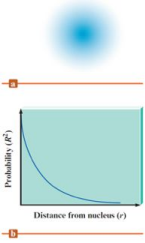● Physical Meaning of Wave Function

○ A radial probability distribution graph plots the total probability of finding an electron in each spherical shell versus the distance from the nucleus

○ (a) Cross section of the hydrogen 1s orbital probability distribution divided into successive thin spherical shells

○ (b) Radial probability distribution plot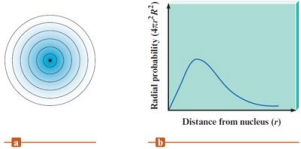○ The size of the 1s orbital can be stated as the radius of the sphere that encloses 90% of the total electron probability

● Quantum Numbers: three parameters in the wave function for the hydrogen atom

numbers

Allowed values

Total number

Determines

orbital’s...

Principal quantum number, n

1,2,3,4...

Size and energy

Angular

momentum

quantum number, l

0 to (n-1)

n

shape

Magnetic

quantum number ml

-1, to +1

2l+1

orientation

○ When n = 1, l = 0;

■ n = 2, l = 0, 1;

■ n = 3, l = 0, 1, 2;

■ n = 4, l = 0, 1, 2, 3

Value of l

0

1

2

3

Letter used

s

p

d

f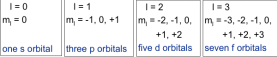● Orbital Shapes and Energies

○ The n determines size and energy of orbital

■ The larger the value of n, the larger the orbital and higher the energy the orbital have

■ The l determines shape of orbital

■ Nodes: areas of zero probability

○ Describing an Orbital

■ Each set of n,l, and ml describes one orbital

■ Orbitals with the same value of n are in the same principal level or principal shell

■ Orbitals with the same values of n and l are in the same sublevel or subshell

■ # of sublevels within a level = n

■ # of orbitals within a sublevel = 2l + 1

■ # of orbitals in a level = n2

● Spin Quantum Number, ms

○ Electron spin: a property of electron that rotates on its axis; there are two possible directions of rotation

○ The spin quantum number has only 2 allowed values: +½ and -½ ○ Pauli Exclusion Principle: no two electrons in the same atom can have the same four quantum numbers

○ Thus, a 1s orbital can “hold” two electrons:

■ Electron 1: n = 1, l = 0, ml = 0, and ms = +½

■ Electron 2: n = 1, l = 0, ml = 0, and ms = -½

○ An orbital can only hold two electrons, and they must have opposite spins because only two values of ms are allowed

● Four Quantum Numbers● Polyelectronic Atoms: atoms with more than one electron

○ Electron correlation problem: since the electron pathways are unknown, electron repulsions cannot be accurately

○ Penetration effect:

■ 3s electron penetrates to the nucleus more than 3p electron

● This causes the 3s electron to be attracted to the nucleus more

strongly than a 3p electron

○ Energy level in polyelectronic atom: Ens < Enp< End < Enf

● Energy splitting in Polyelectronic Atoms

○ For hydrogen atom or single electron systems:

■ Sublevels in each principal all have same energy

■ Orbitals with the same energy are said to be degenerate

○ For polyelectronic atoms

■ Energies of the sublevels are split

● 4s<4p<4d<4f

● Caused by electron-electron interaction

● Early Periodic Table

○ Mendeleev’s periodic table: organized known elements of the time in a table format

● He arranged the rows so that elements with similar

properties would fall in the same vertical columns

● Contained some gaps, which allowed him to predict the

existence and properties of undiscovered elements

● Modern Periodic Table: lists elements in order of atomic number rather than mass

○ Periods: rows

○ Groups: columns

● Electron Configuration: description of the orbitals occupied by electrons ○ Orbital diagram: a square representing each orbital and a half-arrow representing each electron in the orbital

● Rules of Electron Configuration

○ Aufbaus’ principle: electrons ordinarily occupy orbitals of the lowest energy available

■ Energy order: 1s, 2s, 2p, 3s, 3p, 4s, 3d, 4p, etc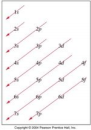● Ex: Li has 3 electrons - 1s22s1

● Ex: Ne has 10 electrons - 1s22s22p6

■ When electrons occupy the lowest energy orbitals the atom is in its ground state

■ When electrons occupy any other orbitals the atom is in an excited state

○ Pauli exclusion principle: one atomic orbital can accommodate only 2 electrons, and these electrons must have opposite spins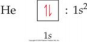○ Hund’s rule: electrons in the same subshell occupy the orbitals singly, with spins parallel, before they are paired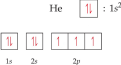○ Condensed electron configuration:

■ (Z = 11) Na: 1s22s22p63s1 Na: [Ne]3s1 1s22s22p6=Ne

■ (Z = 3) Li: 1s22s1 Li: [He]2s1 1s2=H

● Valence Electrons and Core Electrons

○ For main group elements: the electrons in the highest principal energy level called valence electrons - blue

■ Valence electrons are the most important in chemical bonding ○ Electrons in lower energy shells are called core electrons - pink ■ Ex: Si 1s22s22p63s23p2

■ Ge 1s22s22p63s23p64s23d104p2

○ The elements in the same group on the periodic table have the same valence electron configuration and display similar chemical behavior ○ For main group elements - number of valence electrons=group number ● Orbital Blocks on Periodic Table

○ Main group elements:

■ S-block: ns subshell fills

■ P-block: np subshell fills

○ Transition elements:

■ D-block: (n-1)d subshell fills

○ Inner transition elements: f-block: (n-2)f subshell fills● Period Trends in Atomic Properties

○ Atomic radius: one-half of the distance between covalently bonded nuclei

■ Increases in going down a group

● Orbital size increase in successive principal quantum levels ■ Decreases in going across a period from left to right

● Effective nuclear charge increases

● Valence electrons are drawn closer to the nucleus,

decreasing the size of atom

○ Ionization energy(IE): amount of energy required to remove an electron from the ground state of a gaseous atom or ion

■ Requires the input of energy to remove the electron

■ The lower the IE, the easier the electron is to be removed ■ It requires more energy to remove each successive electron ■ When all valence have been removed, IE takes a quantum “leap” ■ 1stionization: Mg (g) Mg+(g) + e-I1 = 735 kJ/mol

2ndionization: Mg+(g) Mg2+(g) + e-I2 = 1445 kJ/mol

3rdionization: Mg2+(g) Mg3+(g) + e-I3 = 7730 kJ/mol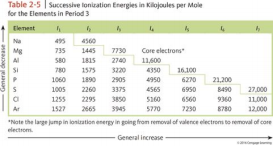■ In general:

● I1 decreases in going down a group

○ The electrons being removed are farther from the nucleus

● increases going left to right

○ Electrons added to the same principal quantum level

cannot completely shield the increasing nuclear charge

and are generally more strongly bound from left to right on

the periodic table

○ Electron affinity(EA): energy change associated with the addition of an electron to a gaseous atom

■ General trend for main group elements:

● Electron affinities become more negative from left to right

across a row

● The halogens have the most negative electron affinities

● Most groups of the periodic table do not exhibit any definite trend in electron affinity

○ Effective nuclear charge (Zeff)

■ A net positive charge attracting a particular electron

■ Increases from left to right across a period

■ Doesn’t change much going down a group

● Metals, Nonmetals, and Metalloids

○ Metals:

■ Low IE, small EA

■ Tend to form cations

○ Nonmetals:

■ High IE, large negative EA

■ Tend to form anions

○ Metalloids:

■ Elements that exhibit both metallic and nonmetallic properties ● Alkali metals

○ Li, Na, K, Rb, Cs, and Fr have low ionization energy, and are the most chemically reactive of the metals

○ Hydrogen exhibits a nonmetallic character due to its small size

Page ExpiredIt looks like your free minutes have expired! Lucky for you we have all the content you need, just sign up here